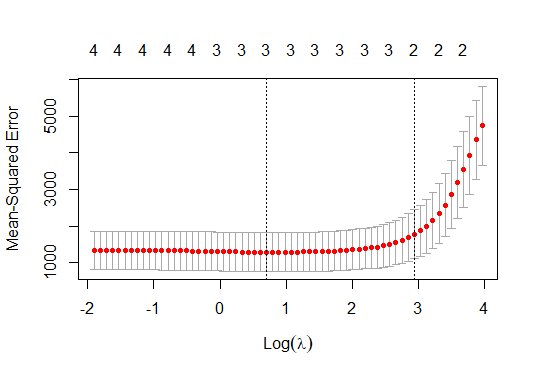# Lasso Regression in R

The Lasso Regression is a classification algorithm that uses the sparse and shrinkage in simple models. This tutorial demonstrates how to perform lasso regression in R.

## Lasso Regression in R

The `LASSO` is abbreviated as Least Absolute Shrinkage and Selection Operator. When we want to automate certain parts of model selection, the lasso regression is a good choice as it shows a high level of multicollinearity.

Linear Regression for Machine Learn...
Linear Regression for Machine Learning | In Detail and Code

The lasso regression is a quadratic programming problem, and the languages like R and Matlab provide solutions. Let’s see step by step process to solve lasso regression in R.

### Understanding the Equation

The Lasso regression minimizes the following function.

``````RSS + λΣ|βj|
``````

Where `j` is the range from 1 to the predictor variable and the `λ ≥ 0`, the second term `λΣ|βj|` is known as shrinkage penalty.

The `RSS = Σ(Yi – ŷi)2`, in which `Σ` is the sum, `yi` is the actual response value for `ith` observation, and `ŷi` is the predicted response value.

The lambda is selected as the lowest possible test mean squad error (MSE) in lasso regression once we know what we will do in the lasso regression.

Let’s load the data in the next step.

### Load the Data

Let’s use the `mtcars` dataset for our example. The `hp` will be used as the response variable and `mpg, drat, wt, qsec` as the predictors.

We can use the `glmnet` package to perform the lasso regression. Let’s load the data.

``````# glmnet package requires to define response variable
x <- mtcars\$hp

# glmnet package requires to define matrix of predictor variables
y <- data.matrix(mtcars[, c('mpg', 'wt', 'drat', 'qsec')])
``````

Once data is loaded, the next is to fit the lasso regression model.

### The Lasso Regression Model Fitting

In this step, we use the `glmnet()` function to fit the lasso regression model; the alpha will be set to 1 for the lasso regression model. The k-fold cross-validation will be performed to determine the value of lambda, and with `glmnet`, it automatically performs cross-validation with `k= 10` folds.

We might need to install the `glmnet` package if it is already not installed. See example:

``````# k-fold cross-validation to find lambda value
cv_model <- cv.glmnet(x, y, alpha = 1)

#lambda value that minimizes test MSE
best_lambda <- cv_model\$lambda.min
best_lambda

# plot the model
plot(cv_model)
``````

The code above fits the lasso regression model and shows the best lambda value and the model plot.

The lambda value which minimizes the test MSE is below.

`````` 2.01841
``````

The lasso regression model plot:### Analyze the Lasso Regression Model

Analyzing the model means we can show to coefficients of the model. The coefficients for our model are:

``````# Coefficients of the model
coef(cv_model)
``````

Output for the code will be:

``````5 x 1 sparse Matrix of class "dgCMatrix"
s1
(Intercept) 418.277928
mpg          -4.379633
wt            .
drat          .
qsec        -10.286483
``````

We know the best lambda value; we can also create the best model bypassing the best lambda value as the argument to the `glmnet` function while fitting the model.

As we can see, no coefficients are shown for the predictors `wt` and `drat` because the lasso regression shrunk them to 0. That is why they were dropped from the model.

## Related Article - R Regression

• Piecewise Regression in R
• Polynomial Regression in R
• Logistic Regression in R